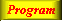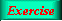Lecture 4 - Page 3 : 34
 Functional Programming in SchemeHigher-order Functions * Introduction to higher-order functions Higher-order functions Some simple and general higher-order functions Linear search in lists Generation of list selectors * Mapping and filtering Classical higher-order functions: Overview Mapping The mapping function Examples of mapping Filtering The filtering function Examples of filtering * Reduction and zipping Reduction The reduction functions Accumulation Zipping The zipping function * Currying The idea of currying Currying in Scheme Examples of currying Ad hoc currying in Scheme (1) Ad hoc currying in Scheme (2) * Web related higher-order functions HTML mirror generation HTML mirror usage examples Making tables with the real mirror Tables with higher-order functions HTML element modifications The function simple-html-table The XHTML mirror in LAML Generation of a leq predicate from enumeration
 Some simple and general higher-order functions It is time to look at some examples of higher-order functions. We start with a number of simple ones.
 ```(define (flip f) (lambda (x y) (f y x)))```

The function flip changes the order of it's parameters. The function takes a function of two parameters, and returns another function of two parameters. The only difference between the input and output function of flip is the ordering of their parameters.An alternative formulation of flip without use of the sugared define syntax.

For easy comparison we show the original version below the alternative formulation. The two different syntaxes are discussed in an earlier lecture, cf. the cross references.

 ```(define (negate p) (lambda (x) (if (p x) #f #t)))```

The function negate negates a predicate. Thus, negate takes a predicate function (boolean function) as parameter and returns the negated predicate. The resulting negated predicate returns true whenever the input predicate returns false, and vise versa.

 ```(define (compose f g) (lambda (x) (f (g x))))```

The function compose composes two functions which both are assumed to take a single argument. The resulting function composed of f and g returns f(g(x)), or in Lisp (f (g x)), given the input x . The compose function from the general LAML library accepts two or more parameters, and as such it is more general than the compose function shown here.Using flip, negate, and compose
 The syntax of function definition